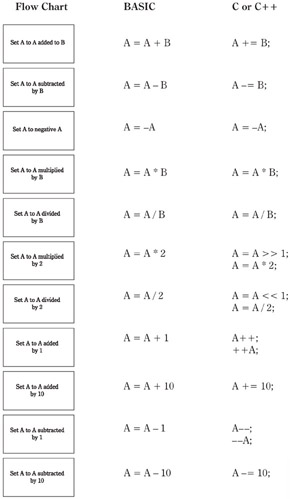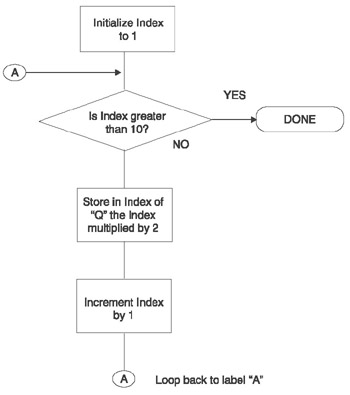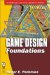# Mathematical StatementsA “loop” statement: Set an array “Q” with ten indexed storage spaces where each index is multiplied by 2.BASIC program for the “loop” flowchart (“'” indicates a comment):

`FOR INDEX = 1 TO 10    'INDEX will start at 1 and end after its                        'increments to 10  Q(INDEX)= INDEX * 2   'Store in index of Q, INDEX multiplied by 2 NEXT INDEX             'Increment INDEX and loop back to FOR`

C or C++ program for the “loop” flowchart (“//” indicates a comment):

`for(INDEX=1; INDEX <= 10; INDEX++) // INDEX will start at 1, check                                     // to see if it’s still less than                                    // or equal to 10 and then                                    // increment INDEX by 1  Q[INDEX] = INDEX * 2;             // Store in index of Q, INDEX                                    // multiplied by 2`

This process can also be initialized as:

`// C starts with index of zero         int Q = { 0, 2, 4, 6, 8, 10, 12, 14, 16, 18, 20};`Game Design Foundations (Wordware Game and Graphics Library)
ISBN: 1556229739
EAN: 2147483647
Year: 2003
Pages: 179

Similar book on Amazon